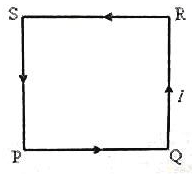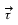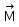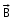NEET  >  Test: Torque on Current Carrying Loop

# Test: Torque on Current Carrying Loop

Test Description

## 10 Questions MCQ Test Physics Class 12 | Test: Torque on Current Carrying Loop

Test: Torque on Current Carrying Loop for NEET 2023 is part of Physics Class 12 preparation. The Test: Torque on Current Carrying Loop questions and answers have been prepared according to the NEET exam syllabus.The Test: Torque on Current Carrying Loop MCQs are made for NEET 2023 Exam. Find important definitions, questions, notes, meanings, examples, exercises, MCQs and online tests for Test: Torque on Current Carrying Loop below.
Solutions of Test: Torque on Current Carrying Loop questions in English are available as part of our Physics Class 12 for NEET & Test: Torque on Current Carrying Loop solutions in Hindi for Physics Class 12 course. Download more important topics, notes, lectures and mock test series for NEET Exam by signing up for free. Attempt Test: Torque on Current Carrying Loop | 10 questions in 10 minutes | Mock test for NEET preparation | Free important questions MCQ to study Physics Class 12 for NEET Exam | Download free PDF with solutions
 1 Crore+ students have signed up on EduRev. Have you?
Test: Torque on Current Carrying Loop - Question 1

### A device which converts electrical energy into mechanical energy is called:​

Detailed Solution for Test: Torque on Current Carrying Loop - Question 1

A device which converts electrical energy into mechanical energy is called an electrical motor.
The working principle of an electric motor depends on the magnetic and electric field interaction.
There are two types of electric motor and they are:
AC motor: Converts alternating current into mechanical power. Linear motor, synchronous motor, and induction motor are the three types of AC motor.
DC motor: Converts direct current into mechanical power. Self-excited motor and separately excited are the two classifications of DC motor.

Test: Torque on Current Carrying Loop - Question 2

### A conductor of length l, carrying current I and placed in a magnetic field B experiences a force F given by

Detailed Solution for Test: Torque on Current Carrying Loop - Question 2

current (i) in a magnetic field ( Bexperiences a force ( Fgiven by the equation

F = i (l × B)

or F = ilB sin θ,

where l is the length of the wire, represented by a vector pointing in the direction of the current.

Test: Torque on Current Carrying Loop - Question 3

### A wire 40 cm long carries a current of 20 A. It is bent into a loop and placed with its plane perpendicular to a magnetic field having a flux density of 0.52 T. What is the torque on the loop if it is bent into a square.​

Detailed Solution for Test: Torque on Current Carrying Loop - Question 3

a2, = I A B
The 40cm of wire can bent into a square with slides of 10cm
A=(10cm)2=100 cm2
τ=I A B
τ=(20A)(100cm2)(0.52T)
τ=1040 A cm2T[1m2/104 cm2]
τ=0.1040 N-m

Test: Torque on Current Carrying Loop - Question 4

A charge moves through a magnetic field with a certain velocity. Direction of force can be obtained by applying :

Detailed Solution for Test: Torque on Current Carrying Loop - Question 4

Solution :- When a charged particle moves in a magnetic field, a force is exerted on the moving charged particle. The formula for the force depends on the charge of the particle, and the cross product of the particle's velocity and the magnetic field.

Test: Torque on Current Carrying Loop - Question 5

A plane square loop PQRS of side ‘a’ made of thin copper wire has ‘n’ turns and it carries a direct current ‘I’ ampere in the direction shown in the adjoining figure. This wire loop is placed in a magnetic field of flux density ‘B’ tesla, which is directed perpendicularly in to the plane of the loop. What is the torque acting on the loop?Detailed Solution for Test: Torque on Current Carrying Loop - Question 5

The magnetic moment of this loop is in direction perpendicular to the plane of loop and coming out of the plane. The magnetic field is also directed perpendicularly into the loop. So, magnetic moment magnetic field are antiparallel. The torque acting on the loop, which is given by the cross product of moment and field, is zero since sin 180 = 0

Test: Torque on Current Carrying Loop - Question 6

Torque on a current loop is by= n(x). With ishere?

Test: Torque on Current Carrying Loop - Question 7

A wire of length l, carrying current is bent into a loop and placed with its plane perpendicular to a magnetic field. In which of the following shapes, is the torque acting on the loop maximum?

Detailed Solution for Test: Torque on Current Carrying Loop - Question 7

The torque on a current loop depends upon the area of the current loop, when the magnetic field is perpendicular to the plane of the loops the torque has its maximum value,
Τ=I A B
We know I and B for all these cases but A depends upon the geometry. The circle has the greatest area so it should provide the greatest torque.

Test: Torque on Current Carrying Loop - Question 8

A current loop of area 0.01m2 and carrying a current of 100 A is held parallel to a magnetic field of intensity 0.1 tesla. The torque in Nm acting on the loop is:

Detailed Solution for Test: Torque on Current Carrying Loop - Question 8

A=0.01m2, I=100A, B=0.1T, θ=90o,sinθ=1
Now magnetic moment M =I×A
Torque, τ=M×B
=MBsinθ
=IAB
=100×0.01×0.1=0.1Nm.

Test: Torque on Current Carrying Loop - Question 9

A coil in shape of an equilateral triangle if side ‘|’ is suspended between the pole pieces of a permanent magnet such that the magnetic field B is in the plane of the coil. If due to a current I in the triangle, a torque Syntax error from line 1 column 49 to line 1 column 73. Unexpected ‘mathsize’. acts on it, the side of the triangle is:

Detailed Solution for Test: Torque on Current Carrying Loop - Question 9

Explanation : The current flowing clockwise in an equilateral triangle has a magnetic field in the direction of k^.

τ = BiNAsinθ

τ = BiNAsin90o

τ = Bi × (√3)/4I2 × 1

[∵ Area of equilateral triangle = (√3)/4I2 and N = 1]

(assuming)

⇒ I2 = 4τ/(3Bi)

=> I = 2[τ/Bi√3]1/2

Test: Torque on Current Carrying Loop - Question 10

A charge of +10Âµ coulomb enters a uniform magnetic field parallel to its direction. What will happen to the charge?​

Detailed Solution for Test: Torque on Current Carrying Loop - Question 10

As the magnetic field is parallel to the charge, no magnetic Lorentz force will act on it and it will move undeviated with constant velocity.

## Physics Class 12

157 videos|452 docs|213 tests
 Use Code STAYHOME200 and get INR 200 additional OFF Use Coupon Code
Information about Test: Torque on Current Carrying Loop Page
In this test you can find the Exam questions for Test: Torque on Current Carrying Loop solved & explained in the simplest way possible. Besides giving Questions and answers for Test: Torque on Current Carrying Loop, EduRev gives you an ample number of Online tests for practice

## Physics Class 12

157 videos|452 docs|213 tests

### How to Prepare for NEET

Read our guide to prepare for NEET which is created by Toppers & the best Teachers